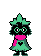#### var text="ralsei butt" var speed=30 // SPEED OF FADE if (document.all||document.getElementById){ document.write('<span id="highlight"><font size="10"><strong>' + text + '</strong></font></span>') var storetext=document.getElementById? document.getElementById("highlight") : document.all.highlight } else document.write(text) var hex=new Array("00","14","28","3C","50","64","78","8C","A0","B4","C8","DC","F0") var r=1 var g=1 var b=1 var seq=1 function changetext(){ rainbow="#"+hex[r]+hex[g]+hex[b] storetext.style.color=rainbow } function change(){ if (seq==6){ b-- if (b==0) seq=1 } if (seq==5){ r++ if (r==12) seq=6 } if (seq==4){ g-- if (g==0) seq=5 } if (seq==3){ b++ if (b==12) seq=4 } if (seq==2){ r-- if (r==0) seq=3 } if (seq==1){ g++ if (g==12) seq=2 } changetext() } function starteffect(){ if (document.all||document.getElementById) flash=setInterval("change()",speed) } starteffect()

• Install GZDoom for your system.

• Make sure that you have a Doom 2 IWAD in the GZDoom folder. (You can get it through "legitimate" means.

• Drag the corresponding files into the GZDoom.exe and enjoy!
• For Dude 2, just drag the .pk3 and .wad into GZDoom and it will run nicely.

• To return to the homepage, click on that little goat on the top!